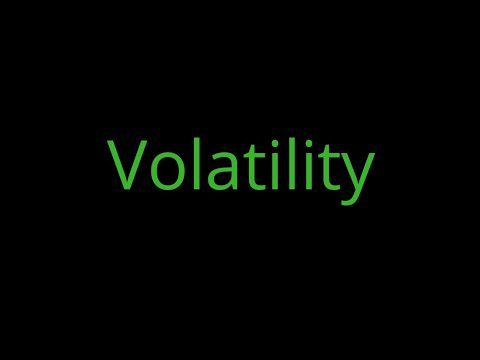## Trending

recent
It is a rate at which the price of a security increases or decreases for a given set of returns. Volatility is measured by calculating the standard deviation of the annualized returns over a given period. It shows the range to which the price of a security may increase or decrease.

Delving into Volatility:

Volatility measures the risk of a security. It is used in option pricing formula to gauge the fluctuations in the returns of the underlying assets. Volatility indicates the pricing behaviour of the security and helps estimate the fluctuations that may happen in a short period.

If the prices of a security fluctuate rapidly in a short time, it is termed to have high volatility. If the prices of a security fluctuate slowly in a longer time, it is termed to have low volatility.

What Implied Volatility Rank?

Implied Volatility Rank is a favoured volatility measure for many option traders. IVR tells us whether implied volatility is high or low in a specific underlying based on the past year of implied volatility data.
For Example, if BataIndia has had IV between 16 and 48 over the past year and IV is currently trading at 28, BataIndia would have an IV rank of 37.5%.
IV Rank is calculated using the formula
IV Rank = ((Current IV - 52-Week IV Low)/(52-Week IV High- 52-Week IV Low))*100
Why Implied Volatility Rank?

Since all underlying have unique IV ranges, stating an arbitrary IV number does not help us decide how we should proceed with a strategy - that's where IVR comes into play.
Understanding where current implied volatility is trading compared to recent history can give a trader a better sense of whether the price of volatility is cheap or expensive given current conditions.

What is Implied Volatility Percentile?

Implied Volatility Percentile (IVP), a totally different calculation, provides traders with another metric by which they can analyze the price of an option. IVP tells us the percentage of days over the last year that implied volatility traded below the current level.

For instance, if IVP is 90%, the understanding is that implied volatility traded below the current level for 90% of the past year’s data. This indicates that implied volatility at the current price is higher than usual.

On the other hand, if IVP is 10%, the understanding is that implied volatility has only traded below current levels 10% of the time over the past year. This second case might indicate that volatility is relatively cheap as compared to the last 12 months of data.

## Purpose of Volatility:

The main idea behind trading is that option sellers drive the market. They sell options and collect premium income in a consistent and high-probability manner. Enabling their portfolio to appreciation steadily month after month without guessing which direction the market will move. The main key for options trading success is leveraging implied volatility and Theta decay to their advantage.
Since options premium pricing is largely determined by implied volatility, it’s IV when used appropriately that provides options traders with a statistical edge over the long-term.
Implied volatility is the market’s prediction of how volatile the stock will be in the future or the expected volatility of a stock. Implied volatility has many implications and relationships that should be grasped.
· The higher the IV, the wider the expected range of the underlying stock movement becomes
· As IV rises, the expectations of share price movement rise and demand for the options increase
· When IV increases, options increase in value
· When IV decreases, options decrease in value

Since option pricing is determined by IV, the option itself will rise and fall as IV or the expectation of volatility changes. IV largely determines whether or not options are relatively cheap or expensive.
The volatility never trends. It swings in a cycle of high and low. Option sellers go Vega short when they find volatility is at the higher end as they know Volatility will cool down so the premium.
What is the difference between Implied Volatility (IV), Implied Volatility Rank (IVR) and Implied Volatility Percentile (IVP) and how to use them in Option TradingReviewed by Kumar Chandan on January 31, 2020 Rating: 5